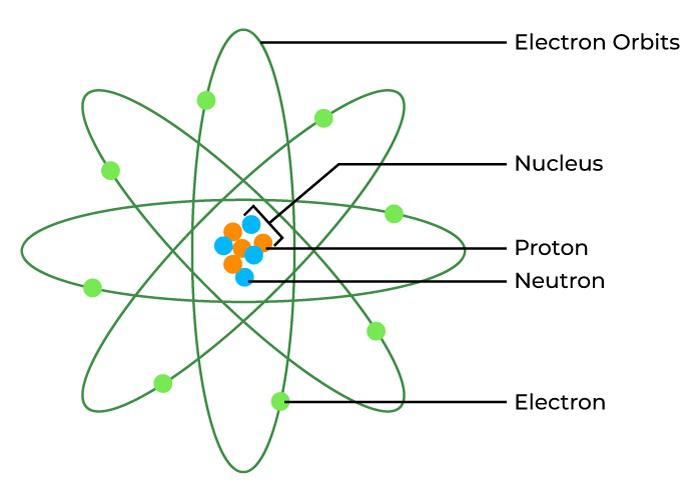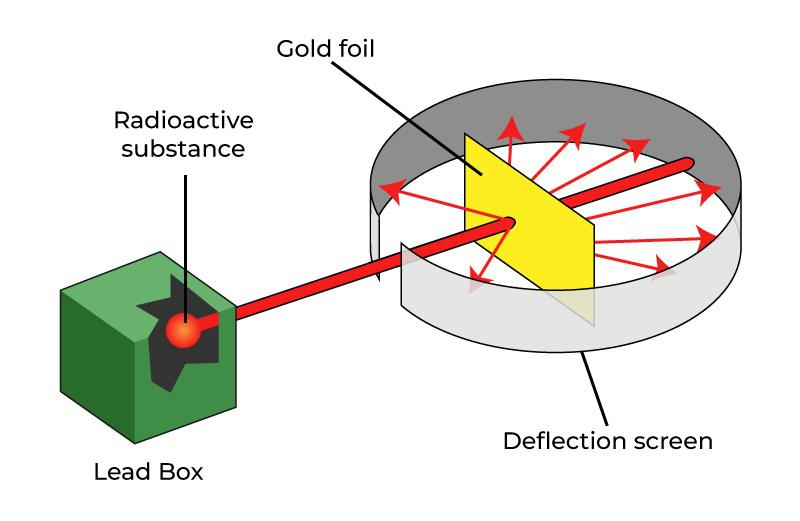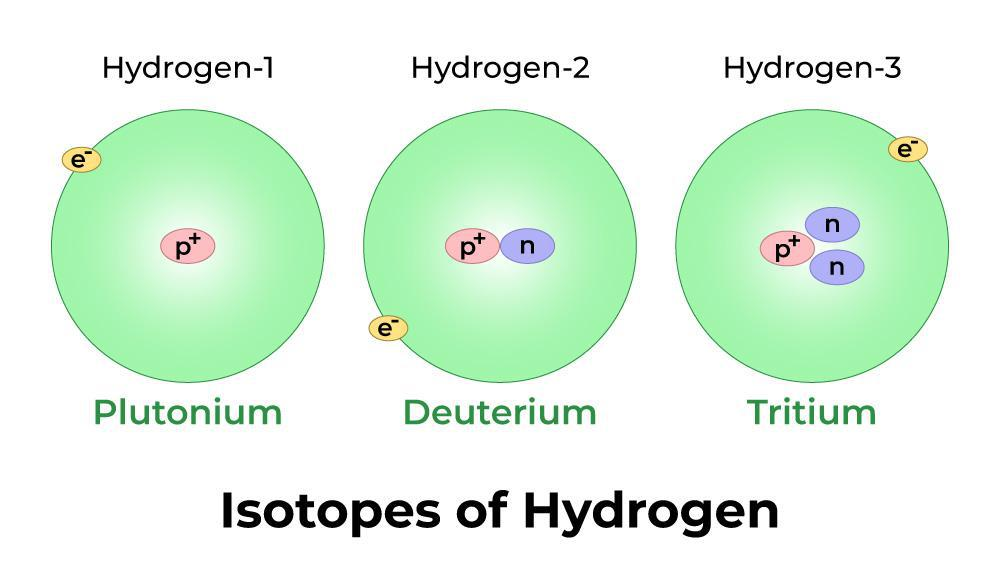Open In App
Related Articles

# Neutrons

Neutrons are fundamental subatomic particles of the atom. An atom is made up of electrons, protons and neutrons. James Chadwick, an English physicist, discovered the neutron in 1932. Neutrons are particles with no charge and higher mass. They are represented by n. They reside inside the nucleus of the atom and along with protons they are called nucleons. Let’s learn about neutrons in detail in this article.

## What is Neutron?

Neutrons are subatomic particles that are one of the primary constituents of atomic nuclei within Electrons and Protons. Neutron, neutral subatomic particle that is a constituent of every atomic nucleus except ordinary hydrogen.

Neutrons and protons are together termed nucleons since they behave in a very similar manner within the nuclei of atoms. The number of nucleons is also called the Mass number. The mass of a nucleon may be roughly approximated to at least one mass unit (often abbreviated to a.m.u.). The branch of science that deals with the study of the properties of neutrons and also the interactions of those subatomic particles with different matter and nonparticulate radiation is named atomic physics.

A neutron is present inside the nucleus of an atom, Hydrogen atom does not contain a nucleus. As protons and neutrons, both are present inside the nucleus, they are combinedly and known as nucleons.

The image given below shows the electrons, protons and neutrons of an atom.## Discovery of Neutron

The existence of neutrons was noted with the help of the Rutherford Nuclear Model of the Atom. there are a few points noted in the discovery of neutrons. (It also gives us remarkable information on the arrangement of the constituent particles)

• In this experiment where most of the alpha particle passes unreflected.
• some of them deviate through smaller angles and some with an angle greater than 180 degrees.
• Thus it indicated the presence of a particle in the middle part of the atom i.e nucleus; it marked the presence of a mass particle and the neutron was discovered later in 1932 by James Chadwick.

The image given below shows the setup for the discovery of Neutrons.As electrons have negligible mass and this means the mass of an atom is only due to the proton only but it would create problems in the model thus it was indicated the presence of some neutral particle with a proton that has equal mass to that of a proton must be present in the atom. Rutherford realized that the atomic mass of different species cannot be determined unless there is the presence of another particle thus, Rutherford in 1920 stated that there is a kind of neutral particle with a mass equal to the mass proton present. James Chadwick, in 1932 during the study of the artificial transmutation of atoms, discovered a particle indicated by Rutherford in 1920. When a  fast-moving alpha particle is bombarded with a thin foil of beryllium and beryllium changes into carbon and emits a neutral particle of the same mass as the proton. This new fundamental particle was named neutron because of its neutral nature and The particle was also found in many other reactions.

## Charge and Mass of Neutron

• A neutron has no electric charge linked with it. Neutrons are neutrally charged subatomic particles as a result.
• A neutron’s mass is approximately 1.008 atomic mass units. The mass of a neutron is approximately 1.674 × 10-27 kg when measured in kilogrammes.
• Because neutrons do not have an electric charge, mass spectrometry cannot be used to estimate their mass directly.
• By subtracting the mass of a proton from the mass of a deuterium nucleus (deuterium is a hydrogen isotope with one proton, one electron, and one neutron in its atomic structure), the mass of the neutron may be computed.
Because the mass of the electron is so little in comparison to the proton and neutron, the mass of the neutron may be estimated by subtracting the proton’s mass from the mass of the deuterium atom.

## Applications of Neutrons

Various applications of Neutrons are,

• Neutrons are highly ionised and penetrating particles that can be employed in boron capture therapy and medical applications. However, when absorbed, they activate the substance and make it radioactive.
• It is significant in nuclear reactions (understanding of the neutron’s behaviour, in particular, has aided in the creation of nuclear weapons and reactors).
• The neutron activation analysis (NAA) method is used to study a simple sample of materials in a nuclear reactor, and the neutron emitter is used to locate light nuclei in the environment.
• It is utilised in medical neutron tomography, but it has unfavourable side effects that leave the afflicted area radioactive, hence it is not frequently employed. The neutron lens was being developed at the time.
• It’s utilised in boron capture treatment, which uses a tiny neutron beam to treat cancer. The energy delivered to malignant regions by neutron radiation is orders of magnitude larger than that delivered by gamma radiation.
• Uranium-235 and plutonium-239 are virtually always utilised in nuclear fission reactors due to nucleus absorption.
• Warm, hot, and cold neutron applications are employed in nuclear scattering facilities where radiation is utilised to condensate matter in X-ray studies.

## Properties of Neutrons

Various properties of Neutrons are,

• The magnetic moment of neutrons is not equal to zero, despite the fact that they are considered neutral particles. Despite the fact that electric fields have no effect on neutrons, magnetic fields have an influence on these subatomic particles.
• Neutrons have a lot of penetration, although not as much as cosmic rays. They haven’t been able to ionise a gas yet.
• The approximate mass of an atom in a.m.u is equal to the entire sum of the neutron and proton present in an atom (atomic mass unit). Its mass is calculated by subtracting the proton’s mass from the atomic mass.
• The magnetic field affects these subatomic particles, whereas an electric field has no influence on them. Its magnetic field is linked to the substructure of quarks and the charge’s internal distribution.
• Neutron is made up of three quarks, which are the fundamental particles that give neutron its magnetic properties.

The table below shows the important other characteristics related to Neutrons,

## Stability of Nucleus

The nucleus of an atom contains neutrons and protons which are combinedly and called nucleons. The nucleus has positively charged particles and protons which tend to repel each other but they reside inside the nucleus due to strong nuclear forces. the stability of neutrons is explained by strong nuclear forces.

A nucleus also has neutrons inside its nucleus and the ratio of protons and neutrons is close to 1:1 if the ratio changes the nucleus becomes unstable and radioactive decay occurs. Weak nuclear forces are responsible for this decay.

Elements having the same number of protons but different numbers of neutrons are called isotopes. Elements with a higher atomic number are radioactive in nature. i.e. their nucleus has radioactive decay.

Example: Hydrogen has three Isotopes and out of which two are radioactive.

The image given below shows the isophote of hydrogen.Also, Check

## Solved Example on Neutrons

Example 1: Calculate the number of neutrons in the sodium atom.

Solution:

Atomic number of sodium (Na) is 11

Mass number of sodium is 23

Thus,

No. of neutrons = Mass Number − Atomic Number

= 23-11

= 12

Example 2: What is the atomic mass of Nitrogen?

Solution:

Let the atomic mass of Nitrogen be a.

Thus,

No. of Neutrons =  Mass Number − Atomic Number

7 =  A – 7

A = 14

Hence, the Atomic Mass of nitrogen is 14.

## FAQs on Neutrons

### Question 1: What is the charge of a Neutron?

Neutrons are neutral particles i.e. they have no or zero charges.

### Question 2: What is the mass of a Neutron?

Neuton is the heaviest subatomic particle and its mass is 1.008 amu or 1.674 × 10-27 kg

### Question 3: What are Nucleons?

Protons and neutrons both are present inside the nucleus, they are combinedly known as nucleons.

### Question 4: How many neutrons does carbon have?

Atomic Number of Carbon (C) is 6.

Mass Number of Carbon is 12.

Thus,

No. of Neutrons =  Mass Number − Atomic Number

= 12 – 6

= 6

Thus, Carbon has 6 neutron

### Question 5: How many neutrons does lithium have?

Atomic Number of Lithium (Li) is 3

Mass Number of Lithium is 7

Thus,

No. of Neutrons =  Mass Number − Atomic Number

= 12 – 6

= 6

Thus, Lithium has 6 neutron